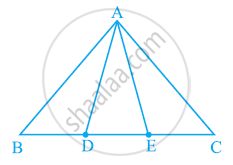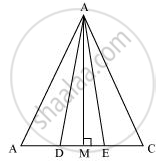Share

# In the following figure, D and E are two points on BC such that BD = DE = EC. Show that ar (ABD) = ar (ADE) = ar (AEC). - Mathematics

Course

#### Question

In the following figure, D and E are two points on BC such that BD = DE = EC. Show that ar (ABD) = ar (ADE) = ar (AEC).

Can you answer the question that you have left in the ’Introduction’ of this chapter, whether the field of Budhia has been actually divided into three parts of equal area?[Remark: Note that by taking BD = DE = EC, the triangle ABC is divided into three triangles ABD, ADE and AEC of equal areas. In the same way, by dividing BC into n equal parts and joining the points of division so obtained to the opposite vertex of BC, you can divide ΔABC into n triangles of equal areas.]

#### Solution

Let us draw a line segment AM ⊥ BC.We know that,

Area of a triangle = 1/2 × Base × Altitude

"Area "(triangleADE)=1/2xxDExxAM

"Area "(triangleABD)=1/2xxBDxxAM

"Area "(triangleAEC)=1/2xxECxxAM

It is given that DE = BD = EC

⇒ 1/2xxDExxAM=1/2xxBDxxAM=1/2xxECxxAM

⇒ Area (ΔADE) = Area (ΔABD) = Area (ΔAEC)

It can be observed that Budhia has divided her field into 3 equal parts.

Is there an error in this question or solution?

#### APPEARS IN

NCERT Solution for Mathematics Class 9 (2018 (Latest))
Chapter 9: Areas of Parallelograms and Triangles
Ex. 9.4 | Q: 2 | Page no. 164

#### Video TutorialsVIEW ALL 

Solution In the following figure, D and E are two points on BC such that BD = DE = EC. Show that ar (ABD) = ar (ADE) = ar (AEC). Concept: Triangles on the Same Base and Between the Same Parallels.
S